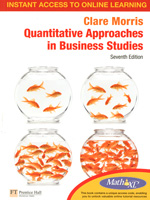Quantitative Approaches in Business Studies,
7th edition

By Clare Morris
Pearson Education
July 2010ISBN: 9780273739418
520 Pages, Illustated
\$99.50 Paper original

Guided Tour
Preface
Acknowledgements
Note to the reader

Introduction: Why quantitative methods?

PART 1: NUMBERS - HOW WE HANDLE THEM
1. Tools of the trade: basic numeracy skills
2. Spreadsheets and other computer-based resources

PART TWO: NUMBERS - A MEANS OF COMMUNICATION
3. Obtaining the figures: data and data collection
4. Presenting the figures: tables and diagrams
5. Summarising the figures: measures of location and spread
6. Measuring changes: index numbers

PART THREE: NUMBERS - A BASIS FOR DEDUCTION
7. A firm foundation: elementary probability
8. Patterns of probability: some distributions
9. Estimating from samples: inference
10. Checking a theory: hypothesis testing
11. Making it better: statistics and quality improvement
12. Looking for connections: correlation
13. Spotting the relationship: line fitting and forecasting
14. Multiple regression

PART 4 NUMBERS - A TOOL OF PLANNING
15. Planning an inventory policy: stock control and simulation
16. Forecasting: time-series, semi-log graphs and exponential smoothing
17. Allowing for interest: financial mathematics
18. Planning production levels: linear programming
19. Planning a project: network analysis
20. Quantitative methods in the student research project

Appendices
1 Suggestions for further reading
2 Random sampling numbers
3 Cumulative binomial probabilities
4 Cumulative Poisson probabilities
5 Areas under the standard normal curve
6 Percentage points of the X2 distribution
7 The correlation coefficient
8 The t-distribution
9 Solutions to selected exercises
Index